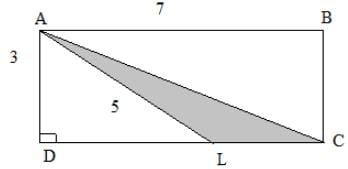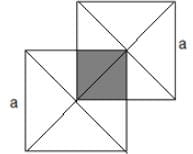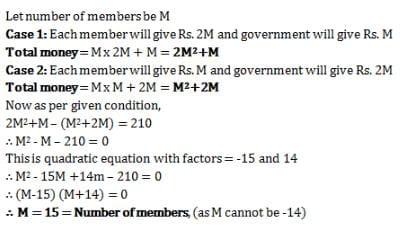# Test: Problem Solving- 1

## 10 Questions MCQ Test Quantitative Reasoning for GMAT | Test: Problem Solving- 1

Description
Attempt Test: Problem Solving- 1 | 10 questions in 20 minutes | Mock test for GMAT preparation | Free important questions MCQ to study Quantitative Reasoning for GMAT for GMAT Exam | Download free PDF with solutions
QUESTION: 1

### What is the area of triangle ALC in the figure given below?Solution:

First of all, we need to find LC, but for that, we need to find DL. We know that AB= DC = 7.

So, LC = DC- DL

Now, DL = √(5^2- 3^2 )= 4 (using Pythagoras Theorem)

So, LC = 7-4 = 3

Therefore, Area = (1/2)(Base)(Altitude) = (0.5)(3)(3) = 4.5

QUESTION: 2

### Alan has two more than twice as many chocolates as does Alice, and half as many chocolates as does Nadia. If Alice has ‘a’ number of chocolates, then in terms of ‘a’, how many chocolates do Alan, Alice and Nadia have?

Solution:

We know that Alice has 'a' chocolates. Alan has 2a+2 chocolates from the given statement in the question. Nadia has double the chocolates as Alan has, so she has 4a+4 chocolates.

Adding these, we get 4a+4+2a+2+a = 7a +6 . So ,Option (d) is correct.

QUESTION: 3

### Milk needs to be thinned to a ratio of 3 parts milk to 2 parts water. The milk-man has by mistake added water so that he has 8 liters of milk which is half water and half milk. What must he add to make the proportions of the mixture correct?

Solution:

We note that the final ratio must be 3:2 for milk and water respectively. Now, according to given scenario in the question, we have eight liters of solution with 4 liters milk and 4 liters water, which makes it 2:2. In order to make it 3:2, we add 2 liters milk. This would make a total of 6 liters milk and 4 liters water i.e. 6:4 which can be simplified to 3:2.

QUESTION: 4

The width of a rectangle is 2/3 times its length. If the length is calculated to be 9, what is the value of perimeter for this rectangle?

Solution:

As the width of the rectangle is 2/3 times its length which is 9, the width comes out to be 6. Therefore, the perimeter becomes, 6+6+9+9 = 30. (Perimeter of a rectangle is calculated by adding the lengths of each side of that rectangle)

QUESTION: 5

A line l is parallel to the y-axis and passes through the point (2,3). What is its gradient (m) and x-intercept?

Solution:

As the given line is parallel to the y-axis, and the slope of y-axis is infinite, therefore its slope is also infinite. As the line is defined by x=2, hence its x intercept will also be 2 because all of its points will be of the form (2,y).

QUESTION: 6

What is the equation of the new parabola created by shifting y = x2 , three units in the positive y-axis direction?

A. y = (x+3)2

B. y = x2

C. y = x2 + 3

D. 3y = x2

E. y = x3

Solution:

As the parabola is shifted above, the change would come in the value of y, so we increase y i.e. the whole function by 3 units. Therefore, y=x2+3

QUESTION: 7

A sphere with diameter 1 unit is enclosed in a cube of side 1 unit each. Find the unoccupied volume remaining inside the cube.

Solution:

We find the volume of the cube by the formula length^3. We then subtract from it the volume of the sphere to find the empty volume. The volume of cube amounts to 1*1*1=1. The volume of the sphere is (4/3)* π *(radius)^3. Putting in the values we get (4/3)* π *(0.5) ^3. This is equal to pi/6. Subtracting the two gives 1- π /6.

QUESTION: 8

The function y=3x2 is shifted 2 units towards the positive x-axis (right) and 3 units towards the positive y-axis (up). Find the resulting function.

A. y=3x2+5

B. y=3x2

C. y=3(x+2)2+3

D. y=3(x-2)2-3

E. y=3(x-2)2+3

Solution:

The upward shift is simply addressed by adding three in the given function, and the horizontal shift is addressed by changing the value of x in the given function. If the shift is a units towards right, then we replace x with x-a. If the shift is towards left then we replace x with x+a.

QUESTION: 9

Find the shaded area when two squares with side ‘a’ intersect as shown in the figure below.A. 1/8 a2

B. 1/4 a2

C. a2

D. 1/3 a2

E. 2/5 a2

Solution:

We realize that the shaded figure is actually a square of side 0.5a. The answer follows, area=0.5a*0.5a=0.25a^2. (area of a square of side a is a^2)

QUESTION: 10

A group wanted to renovate their club. Each member contributed an amount equal to twice the number of members in the club. But the government contributed same amount as the number of members. If each member had contributed the same amount as the number of members and the government had given an amount twice the number of members, then they would have Rs. 210 less. How many members are there?

Solution:Use Code STAYHOME200 and get INR 200 additional OFF Use Coupon Code